# Balancing Equations Balancing Writing and Naming Equations Balancing

• Slides: 34Balancing Equations Balancing, Writing, and Naming Equations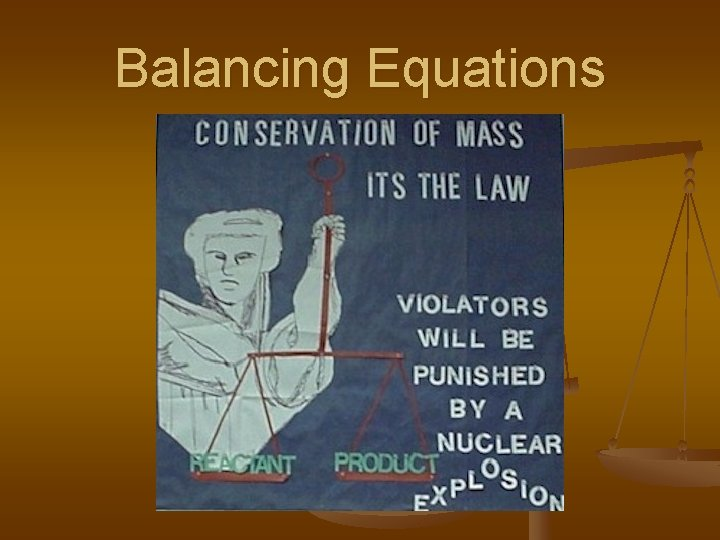Balancing EquationsBalancing Equations n Law of Conservation of Matter: n In a chemical reaction, matter can be neither created nor destroyed. n In a chemical reaction, the amount of reactants equal the amount of products. nBalancing Equations Paraphrase: n Law of Conservation of Atoms: n n The number of atoms of each type of element must be the same on each side of the equation.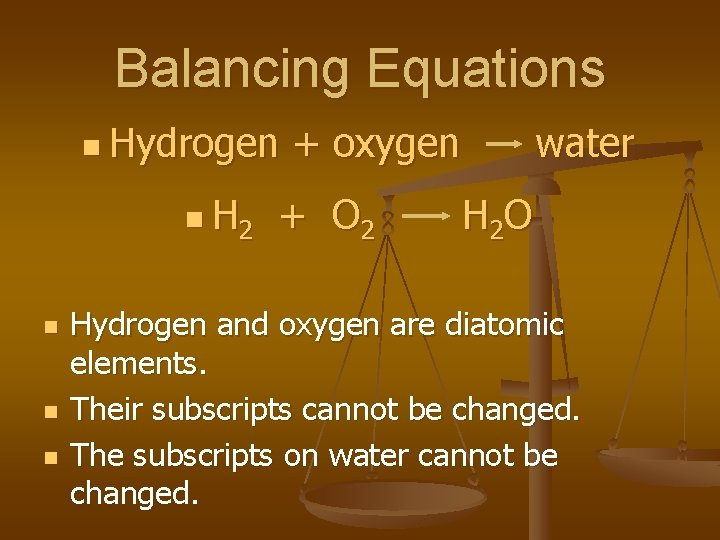Balancing Equations n Hydrogen n H 2 n n n + oxygen + O 2 water H 2 O Hydrogen and oxygen are diatomic elements. Their subscripts cannot be changed. The subscripts on water cannot be changed.Balancing Equation n H 2 n + O 2 H 2 O Count the atoms on each side. n Reactant side: 2 atoms H and 2 atoms O n Product side: 2 atoms H and 1 atom OBalancing Equations n H 2 n n + O 2 H 2 O If the subscripts cannot be altered, how can the atoms be made equal? Adjust the number of molecules by changing the coefficients.Balancing Equations n H 2 + O 2 2 H 2 O Reactants: 2 atoms of H and 2 atoms of O n Products: 4 atoms of H and 2 atoms of O n H is no longer balanced! nBalancing Equations n 2 H 2 n n n + O 2 2 H 2 O Reactant side: 4 atoms of H and 2 atoms of O Product side: 4 atoms of H and 2 atoms of O It’s Balanced!Balancing Equations n N 2 n n + H 2 Nitrogen + hydrogen NH 3 ammonia Count atoms. n Reactants: 2 atoms N and 2 atoms H n Products: 1 atom N and 3 atoms of NH 3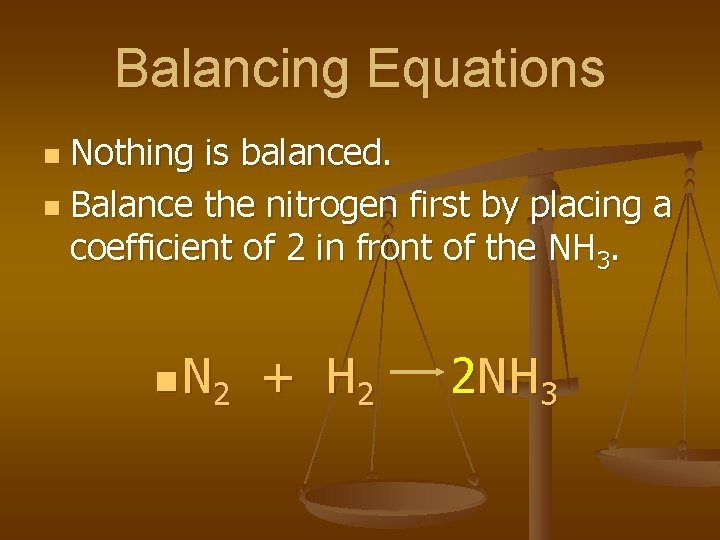Balancing Equations Nothing is balanced. n Balance the nitrogen first by placing a coefficient of 2 in front of the NH 3. n n N 2 + H 2 2 NH 3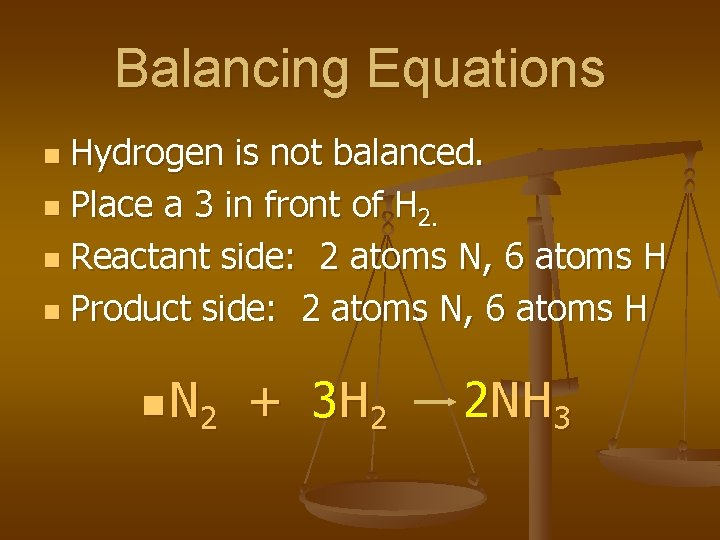Balancing Equations Hydrogen is not balanced. n Place a 3 in front of H 2. n Reactant side: 2 atoms N, 6 atoms H n Product side: 2 atoms N, 6 atoms H n n N 2 + 3 H 2 2 NH 3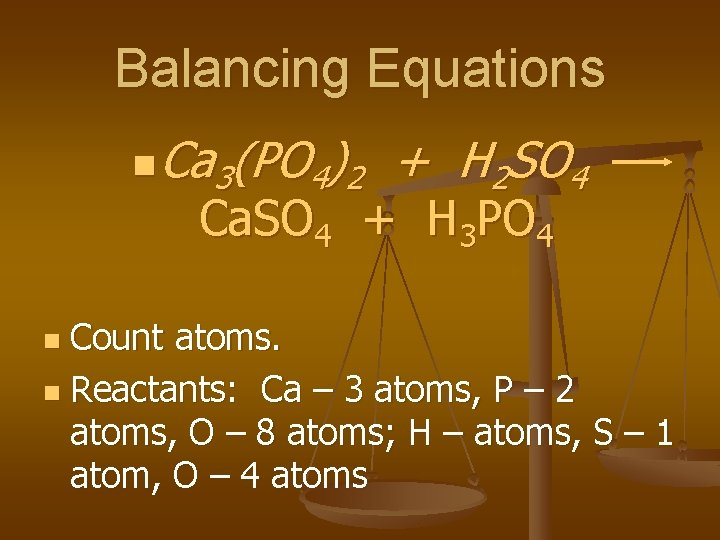Balancing Equations n Ca 3(PO 4)2 + H 2 SO 4 Ca. SO 4 + H 3 PO 4 Count atoms. n Reactants: Ca – 3 atoms, P – 2 atoms, O – 8 atoms; H – atoms, S – 1 atom, O – 4 atoms n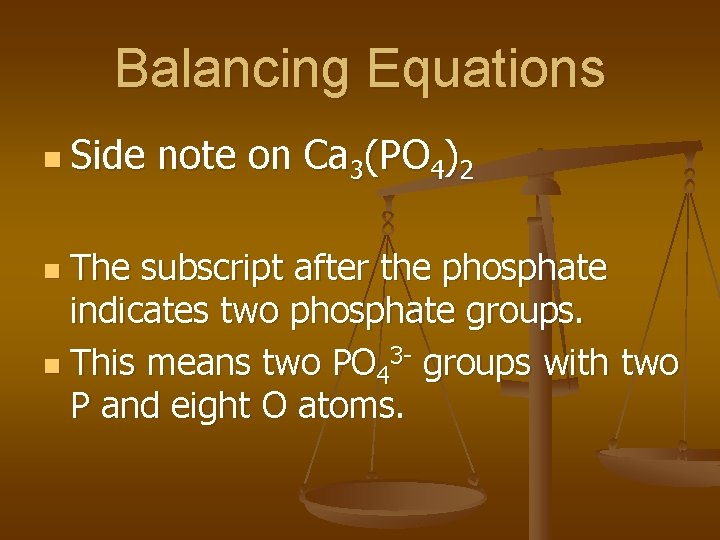Balancing Equations n Side note on Ca 3(PO 4)2 The subscript after the phosphate indicates two phosphate groups. n This means two PO 43 - groups with two P and eight O atoms. nBalancing Equations n Ca 3(PO 4)2 + H 2 SO 4 Ca. SO 4 + H 3 PO 4 n n Count atoms in the product. Ca atoms – 1, S atom – 1, O atoms – 4; H atoms – 3, P atom – 1, O atoms - 4Balancing Equations n n In this equation, the ion groups do not break up. Instead of counting individual atoms, ion groups may be counted. n Ca 3(PO 4)2 + H 2 SO 4 Ca. SO 4 + H 3 PO 4Balancing Equations n Ca 3(PO 4)2 + H 2 SO 4 Ca. SO 4 + H 3 PO 4 n n Reactants: Ca 2+ – 3, PO 43 - - 2, H+ – 2, SO 42+ - 1 Products: Ca 2+ - 1, SO 42 - - 1, H+ - 3, PO 43 -1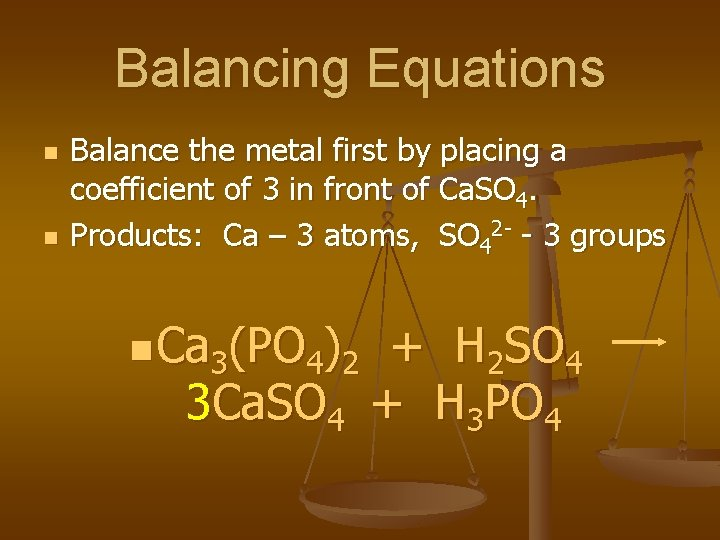Balancing Equations n n Balance the metal first by placing a coefficient of 3 in front of Ca. SO 4. Products: Ca – 3 atoms, SO 42 - - 3 groups n Ca 3(PO 4)2 + H 2 SO 4 3 Ca. SO 4 + H 3 PO 4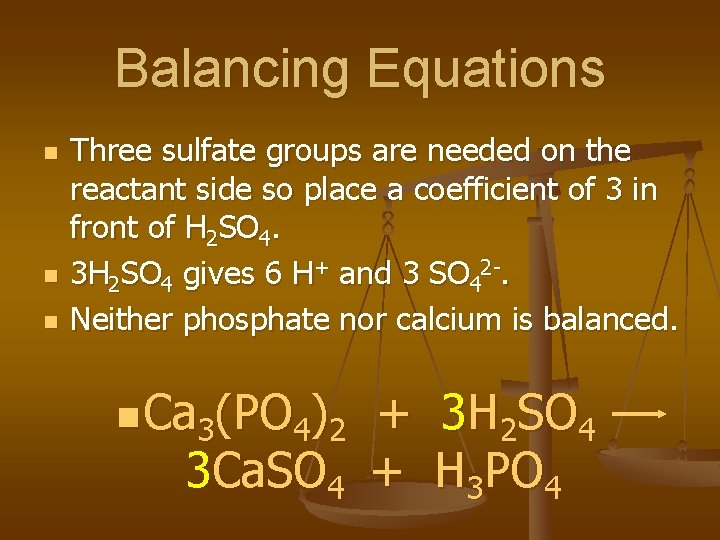Balancing Equations n n n Three sulfate groups are needed on the reactant side so place a coefficient of 3 in front of H 2 SO 4. 3 H 2 SO 4 gives 6 H+ and 3 SO 42 -. Neither phosphate nor calcium is balanced. n Ca 3(PO 4)2 + 3 H 2 SO 4 3 Ca. SO 4 + H 3 PO 4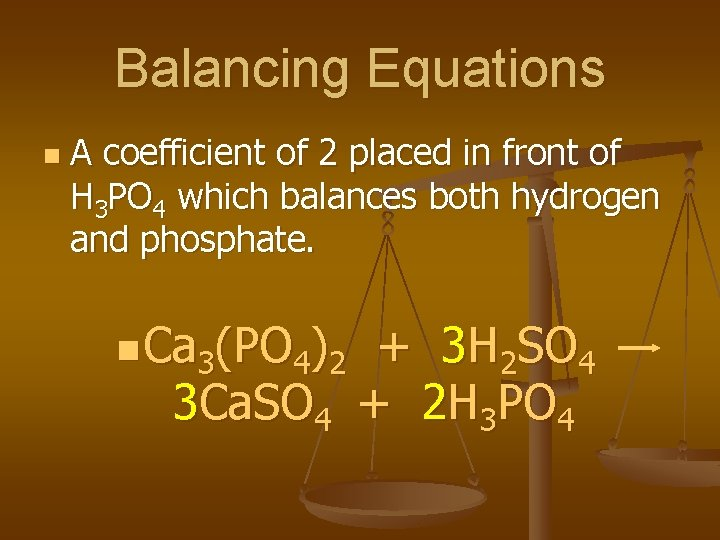Balancing Equations n A coefficient of 2 placed in front of H 3 PO 4 which balances both hydrogen and phosphate. n Ca 3(PO 4)2 + 3 H 2 SO 4 3 Ca. SO 4 + 2 H 3 PO 4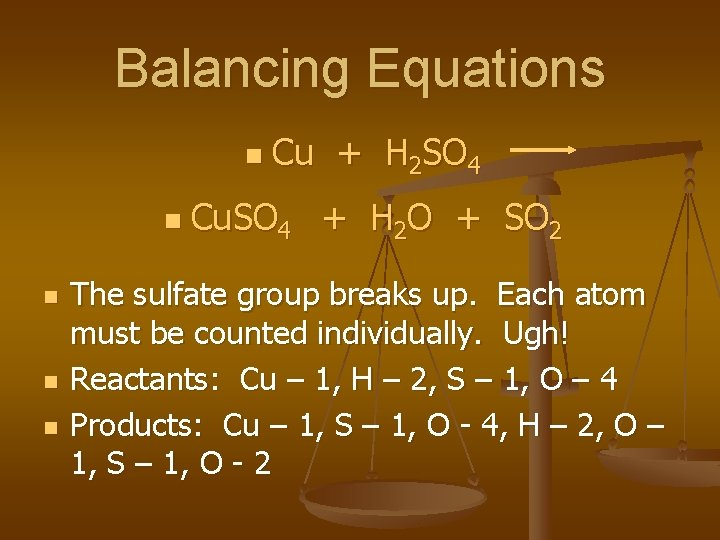Balancing Equations n n n Cu + H 2 SO 4 Cu. SO 4 + H 2 O + SO 2 The sulfate group breaks up. Each atom must be counted individually. Ugh! Reactants: Cu – 1, H – 2, S – 1, O – 4 Products: Cu – 1, S – 1, O - 4, H – 2, O – 1, S – 1, O - 2Balancing Equations Sulfur is not balanced. n Place a two in front of sulfuric acid. n Count atoms: 2 H 2 SO 4 H – 4, S – 2, O-8 n Cu + 2 H 2 SO 4 n Cu. SO 4 + H 2 O + SO 2 nBalancing Equations Hydrogen needs to be balanced so place a 2 in front of the H 2 O. n Count the number of atoms. n Cu + 2 H 2 SO 4 n Cu. SO 4 + 2 H 2 O + SO 2 n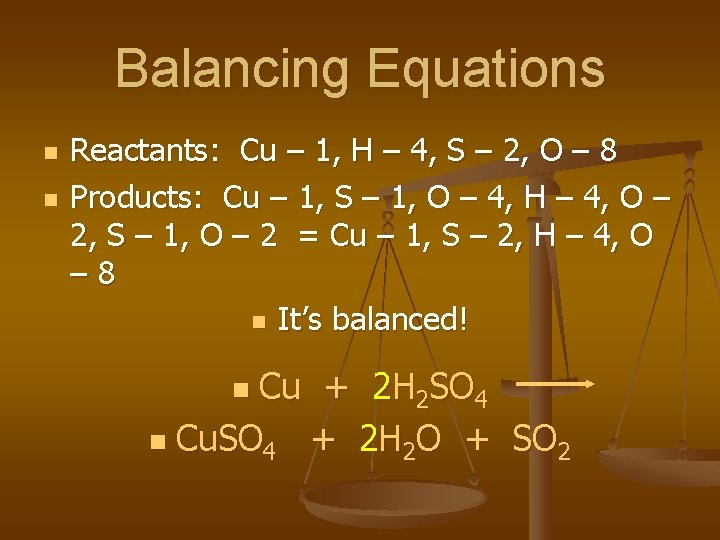Balancing Equations n n Reactants: Cu – 1, H – 4, S – 2, O – 8 Products: Cu – 1, S – 1, O – 4, H – 4, O – 2, S – 1, O – 2 = Cu – 1, S – 2, H – 4, O – 8 n It’s balanced! Cu + 2 H 2 SO 4 n Cu. SO 4 + 2 H 2 O + SO 2 n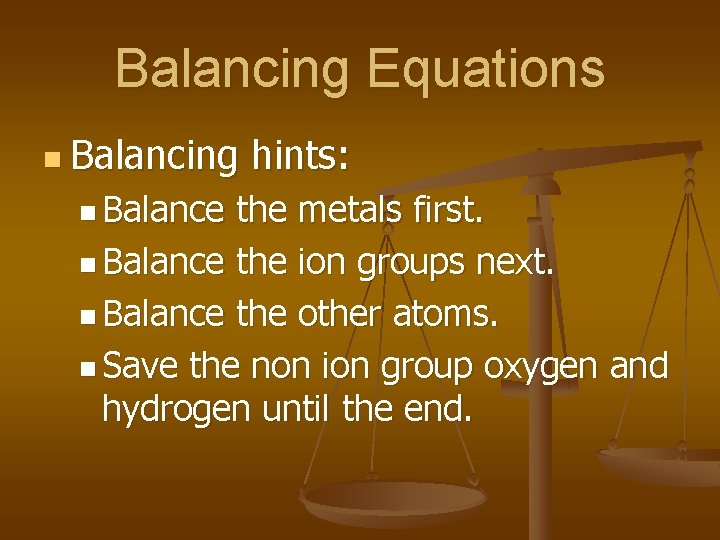Balancing Equations n Balancing n Balance hints: the metals first. n Balance the ion groups next. n Balance the other atoms. n Save the non ion group oxygen and hydrogen until the end.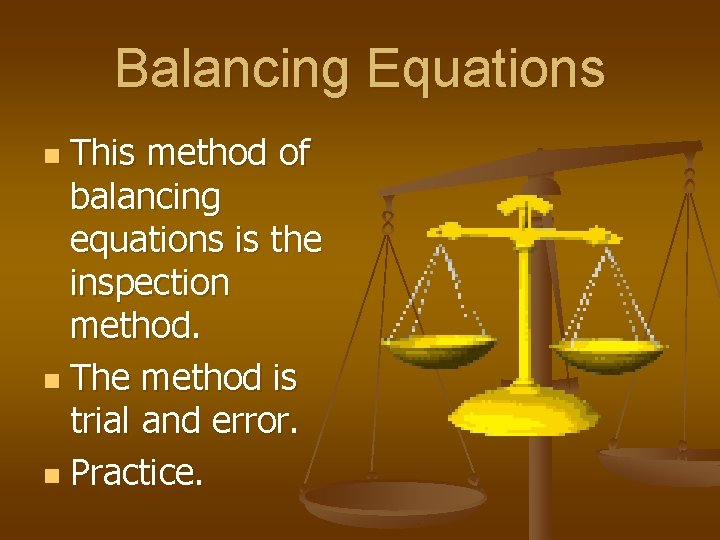Balancing Equations This method of balancing equations is the inspection method. n The method is trial and error. n Practice. nWriting and Naming n n n Nickel + hydrochloric acid Nickel(II) chloride + hydrogen Write the corresponding formula equation and then balance the equation.Writing and Naming n n n Write each formula independently. Ignore the rest of the equation. Balance the equation after writing the formulas. Ni + HCl Ni. Cl 2 + H 2 Ni + 2 HCl Ni. Cl 2 + H 2 n nWriting and Naming n Remember the diatomic elements: H 2, N 2, O 2, F 2, Cl 2, Br 2, and I 2.Writing and Naming Cu + H 2 SO 4 n Cu. SO 4 + H 2 O + SO 2 n Balance the formula equation. n Write the word equation. n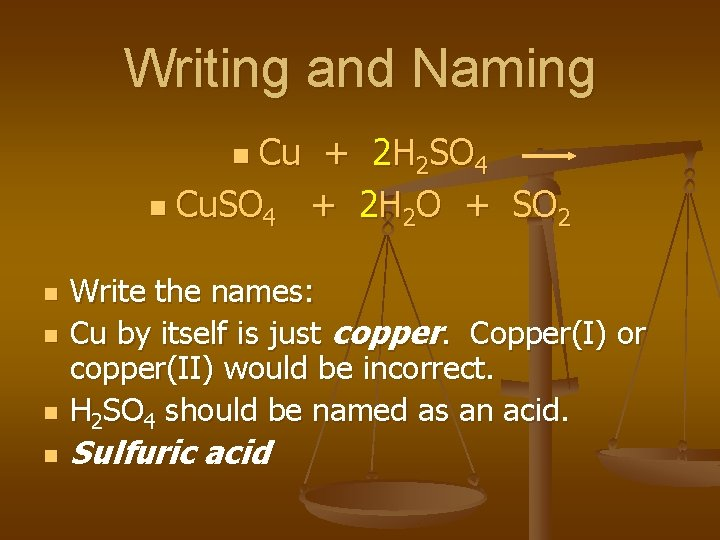Writing and Naming Cu + 2 H 2 SO 4 n Cu. SO 4 + 2 H 2 O + SO 2 n n n Write the names: Cu by itself is just copper. Copper(I) or copper(II) would be incorrect. H 2 SO 4 should be named as an acid. Sulfuric acidWriting and Naming n Cu. SO 4 has a SO 42 - group so Cu must be 2+. Some metals must have Roman Numerals. Copper(II) sulfate H 2 O is known as water. n SO 2 is a nonmetal compound. Its name is either sulfur dioxide or sulfur(IV) oxide. nWriting and Naming Cu + 2 H 2 SO 4 n Cu. SO 4 + 2 H 2 O + SO 2 n Copper + sulfuric acid n Copper(II) sulfate + water + sulfur dioxide nBalancing Equations n Chapter 8 n Practice!